﻿ 强化学习的地–空异构多智能体协作覆盖研究
«上一篇文章快速检索 高级检索

 智能系统学报2018, Vol. 13Issue (2): 202-207  DOI: 10.11992/tis.2016090170

### 引用本文ZHANG Wenxu, MA Lei, HE Huilin, et al. Air-ground heterogeneous coordination for multi-agent coverage based on reinforced learning[J]. CAAI Transactions on Intelligent Systems, 2018, 13(2): 202-207. DOI: 10.11992/tis.201609017.### 文章历史

Air-ground heterogeneous coordination for multi-agent coverage based on reinforced learning
ZHANG Wenxu, MA Lei, HE Huilin, WANG Xiaodong
School of Electrical Engineering, Southwest Jiaotong University, Chengdu 610031, China
Abstract: With the heterogeneous coordinate task of unmanned aerial vehicles (UAVs) and unmanned ground vehicle (UGVs) as the background to this study, a novel air-ground heterogeneous coverage model for a coordinated multi-agent is proposed by the complementation between UAV and UGV heterogeneity, in order to extend and improve the dynamic coverage of a heterogeneous multi-agent system. During the coverage process, the advantages of mobility and the observation scope of the UAV were used in order to guide the actions of the UGV. Moreover, in view of the partial agent observability and uncertainty, decentralized and partially observable Markov decision processes (DEC-POMDPs) were applied as the model in order to establish the coverage environment. Additionally, the reinforced learning algorithm of multi-agents was utilized in order to complete the coverage of the environment. The simulation results revealed that the coverage process was accelerated by the cooperation of the UAV and UGV. Additionally, the reinforced learning algorithm also improved the effectiveness of the coverage model.
Key words: heterogeneous multi-agent system    coverage    air-ground    UAV/UGV    DEC-POMDPs    reinforced learning

1 问题描述 1.1 多智能体覆盖问题

1.2 分布式马尔可夫模型

 $\left\langle {I, S, \left\{ {{A_i}} \right\}, P, \left\{ {{\varOmega _i}} \right\}, O, R, {b^0}} \right\rangle$ (1)

1.3 Q学习

 ${Q^*}(s, a) = R(s, a) + \gamma \sum\nolimits_{s' \in S} {P(s, a, s')} \max {Q^*}(s', a')$ (2)

2 覆盖问题设计 2.1 异构多智能体设计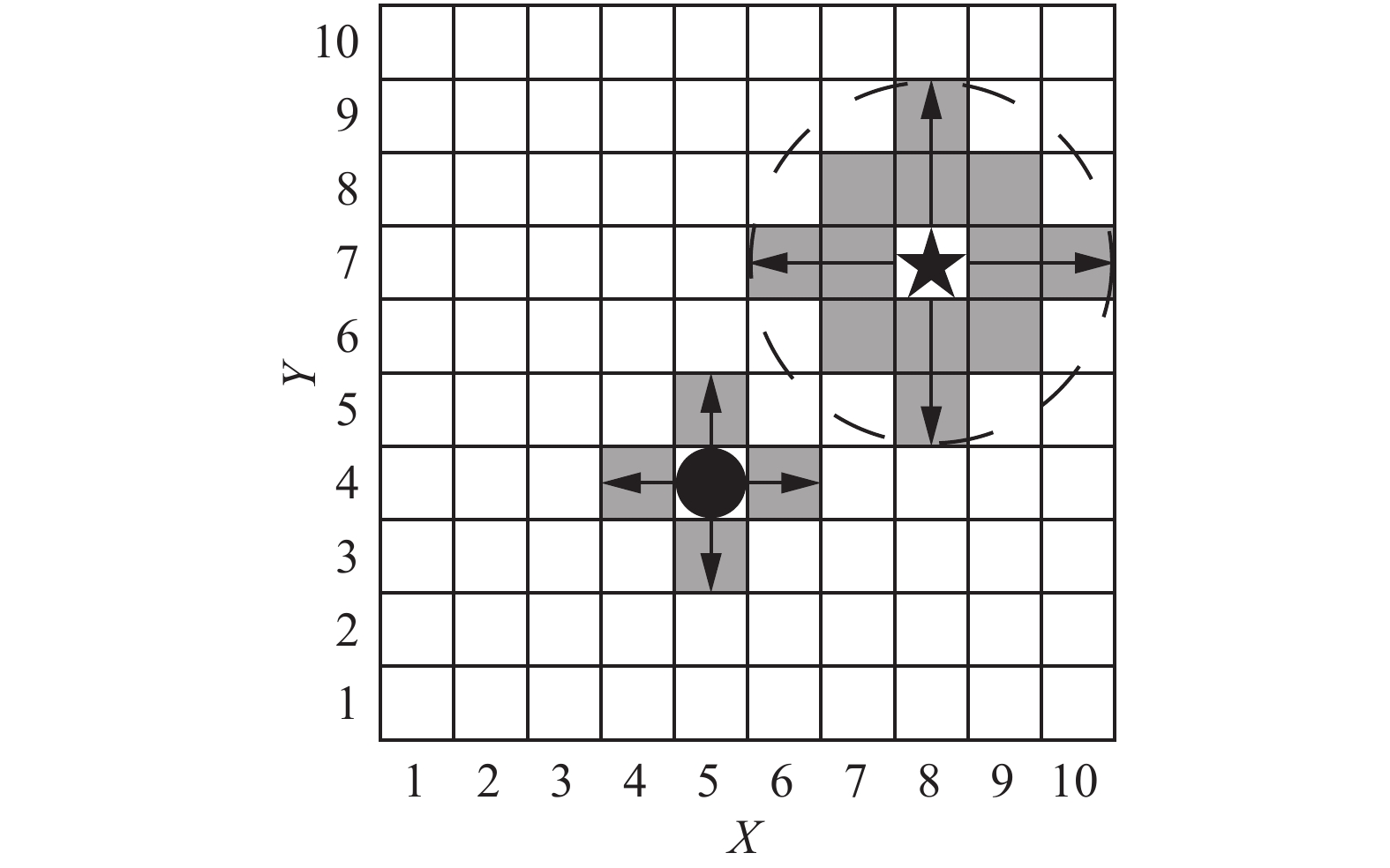Download: 图 1 UAV and UGV的异构观测 Fig. 1 The heterogeneous observation of UAV and UGV

UAV获得的观测信息不仅用于决策UAV的下一步移动动作，还需要向UGV提供额外的地图环境信息。其次，考虑到UAV的速度异构特性，定义其移动速度为每步2个栅格，图中箭头表示智能体的移动方向。UGV以圆圈表示，不同于UAV具备广阔的高空视野，UGV的观测范围较小，定义其观测为前、后、左、右4个栅格，即周围的阴影栅格，设定移动速度为每步1个栅格。UGV的优势在于对环境信息的测量精度要高于UAV。

2.2 覆盖场景设计

$I$ 为智能体数量集合： $I = \left\{ {1, 2, 3} \right\}$ ，异构多智能体系统包含3个智能体，其中编号为1和2的智能体为UGV，编号为3的智能体是UAV。

${{S}}$ 为状态矩阵：用来描述整张地图上各栅格被访问的情况，即各智能体自身的状态，状态集合为 ${{S}}:J \times L$ 。其中 $J$ 表示地图被覆盖的情况，地图上每个栅格的状态信息又可表示为尚未访问状态 ${s_1}$ 、已访问状态 ${s_2}$ 、障碍物状态 ${s_3}$ 3类情况。 $L$ 包含第 $i$ 个智能体在地图上的位置 ${P_i}$

${\varOmega _i}$ 为观测集合：表示第 $i$ 个智能体的观测集合。对UGV而言， ${\varOmega _i}\left( t \right) = \left\{ {{{{env}}_i}\left( t \right), {{po}}{{{s}}_i}\left( t \right), {{po}}{{{s}}_j}\left( t \right)} \right\}$ , $i \in \left[{1, 2} \right]$ ，依次描述 $t$ 时刻第 $i$ 个UGV的自身局部观测信息 ${{{env}}_i}\left( t \right)$ 、自身的位置信息 ${{{pos}}_i}\left( t \right)$ 以及根据通信获得的其他UGV位置信息 ${{{pos}}_j}\left( t \right)$ ，设定UGV无法观测到UAV的位置；对于UAV而言，观测集合 ${\varOmega _3}\left( t \right) =$ $\left\{ {{{{env}}_3}\left( t \right), {{{pos}}_1}\left( t \right), {{{pos}}_2}\left( t \right), {{{pos}}_3}\left( t \right)} \right\}$ ，依次描述 $t$ 时刻UAV自身对环境的观测、各个UGV的相对位置(当UGV处于UAV传感范围时)、UAV自身的位置。在环境观测矩阵 ${{{env}}_i}\left( t \right)$ 中，包含智能体观测范围内 $n$ 个点的环境观测信息集合 $j\left( t \right) = \left\{ {{\varepsilon _1},{\varepsilon _2},} \right.$ $\left. {{\varepsilon _3},{\varepsilon _4}} \right\}$ , 其中 ${\varepsilon _1}$ 表示尚未访问的栅格， ${\varepsilon _2}$ 表示已经访问的栅格， ${\varepsilon _3}$ 表示障碍物栅格， ${\varepsilon _4}$ 表示UGV。智能体的传感器感应范围内出现其他UGV时，能根据该UGV对环境的状态感知获取其相对位置，对于一辆UGV而言，其观测范围内的其他UGV，作为障碍物进行考虑。

$O$ 为观测概率函数：体现了智能体观测的不确定性， $O\left( {o|s, a} \right)$ 表示智能体执行 $a$ 后转移到状态 $s$ 时获得观测 $o$ 的概率。智能体从所处环境中获取观测信息可以用概率矩阵进行表示，假设栅格地图上观测点的观测函数相同，定义其观测–状态概率分布函数如表1所示。表 1 观测-状态概率分布函数 Tab.1 The probability distribution function of observation-state

$O\left( {{s_1}|{\varepsilon _1}, a} \right) = 0.9$ 表示在执行 $a$ 后，到达真实状态 ${s_1}$ 时， ${s_1}$ ${\varepsilon _1}$ 的观测概率为0.9。

${A_i}$ 为动作集合：表示第 $i$ 个智能体的动作。对于UGV和UAV， $t$ 时刻可能产生的动作为 ${A_i}\left( t \right) =\{ {up},$ ${{{ down, left, right}}} \}$

$R$ 为回报函数：表示环境对智能体的行动给出的评价。对于UGV，执行一次行动后存在着“没走过”、“走过”和“障碍物”3个状态，分别对应着30、–5和–10的回报值，栅格的边界作为障碍物考虑。在覆盖问题中，UAV对UGV的观测起指导作用，所以UAV的回报由两部分组成，第1部分为UAV自身的回报，和UGV的回报定义相同，第2部分为UGV反馈的回报，其定义为

 ${R_{{UAV}}} = \mu \cdot {R_{{UAV}}} + \left( {1 - \mu } \right) \cdot \sum {{R_{{UGV}}}}$ (3)

${b^0}$ 为初始信念状态：智能体根据初始信念状态和初始Q值函数获取当前应选择的动作向量。其更新公式为

 ${b^t}\left( {s\left( {t + 1} \right)} \right) = O\left( {{s_1}|\varepsilon, a} \right)\sum\limits_{s \in S} {{b^t}\left( {s\left( t \right)} \right)} P\left( {s\left( {t + 1} \right)|s\left( t \right), a} \right)$ (4)
3 基于强化学习的覆盖算法 3.1 异构多智能体学习决策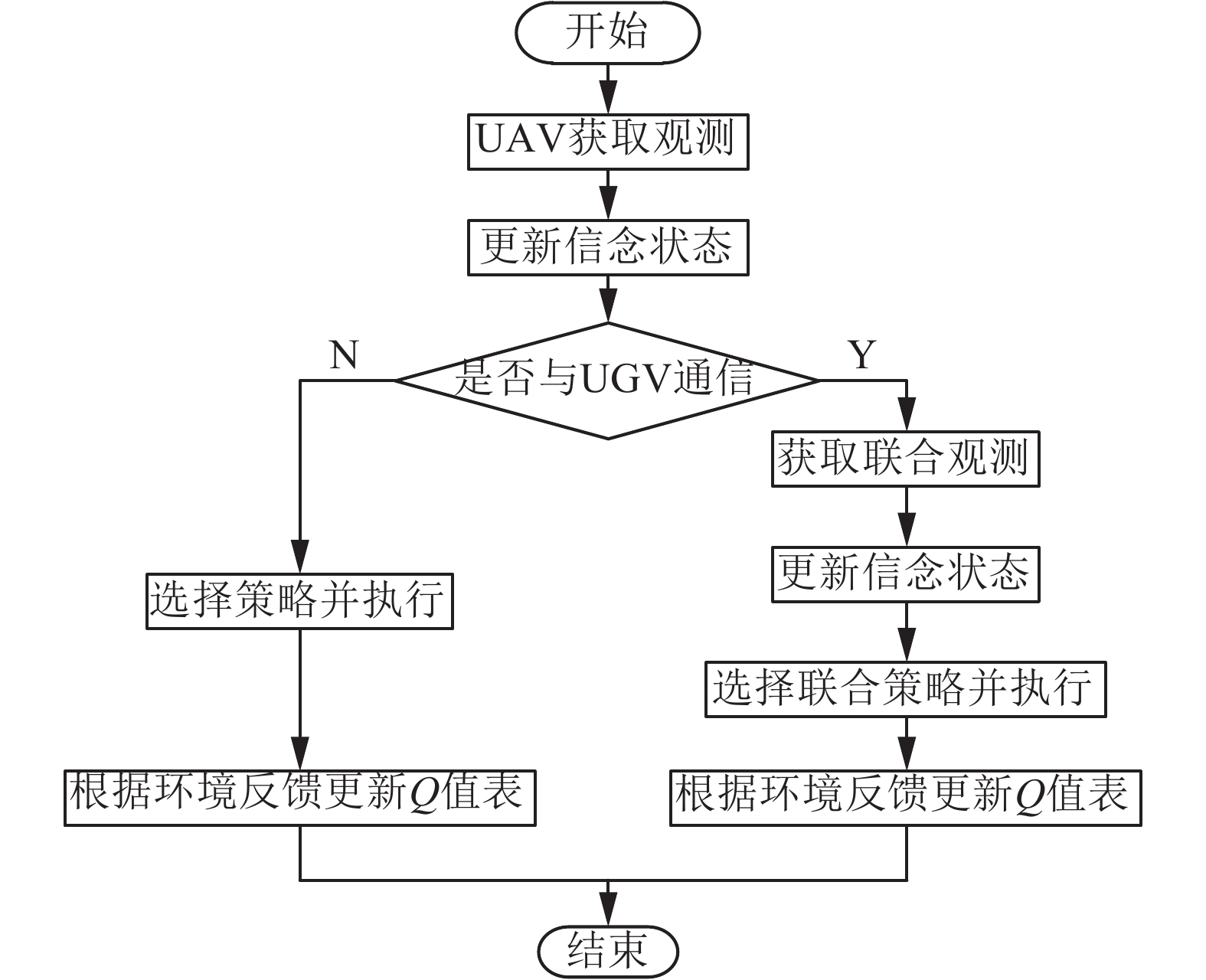Download: 图 2 UAV强化学习一步策略更新流程 Fig. 2 The one-step strategy update flow of reinforcement learning of UAV

UGV获得的观测能够被分为两类：1)根据智能体自身传感器获得的局部观测信息 ${\varOmega _{{{local}}}}$ ；2)依赖通信行为获得的UAV的观测信息 ${\varOmega _{{{other}}}}$ ，则联合观测表示为 ${\varOmega _{{{joint}}}} = \{ {\Omega _{{{local}}}},{\Omega _{{{other}}}} \cup$ Ø}， ${\varOmega _{{{local}}}} \in {\varOmega _{{{joint}}}}$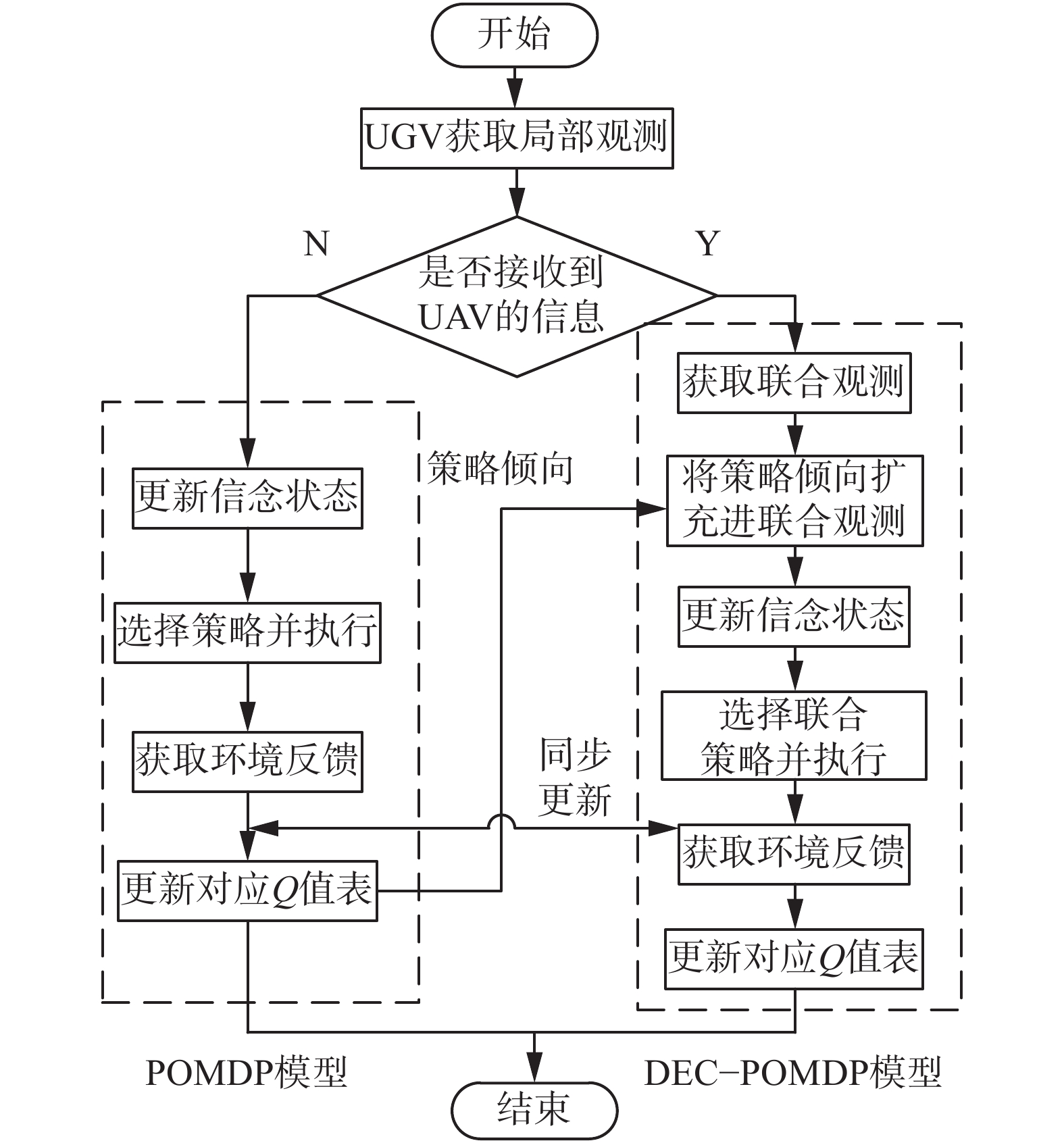Download: 图 3 异构多智能体强化学习框架 Fig. 3 The frame of reinforcement learning of heterogeneous multi-agent

3.2 基于强化学习的覆盖算法

 $\begin{array}{c}{Q_i}\left( {s(t),a} \right) = R\left( {s(t),a} \right) + \\ \sum\limits_{s \in S} {\sum\limits_{o \in \varOmega } {P\left( {s(t + 1)\left| {s(t),a} \right.} \right)O\left( {o\left| s \right.(t + 1),a} \right)V\left( {s(t + 1),a} \right)} } \end{array}$ (5)

Q学习更新公式为

 $\begin{array}{c}{Q_t}\left( {s(t),a} \right) = (1 - \alpha ){Q_t}\left( {s(t),a} \right) + \\ \alpha \left[ {R\left( {s(t),a} \right) + \mathop {\max }\limits_a \left\{ {{Q_t}\left( {s(t + 1),a} \right)} \right\}} \right]\end{array}$ (6)

DEC-POMDPs与POMDP的唯一区别在于智能体的数量由单个变为多个，其Q-学习迭代表达式与POMDP类似，智能体的行动由单独行动 $a$ 变为联合行动 ${{a}}$

 $\begin{array}{c}{Q_t}\left( {s(t),{{a}}} \right) = (1 - \alpha ){Q_t}\left( {s(t),{{a}}} \right) + \\ \alpha \left[ {R\left( {s(t),{{a}}} \right) + \mathop {\max }\limits_a \left\{ {{Q_t}\left( {s(t + 1),{{a}}} \right)} \right\}} \right]\end{array}$ (7)
4 仿真结果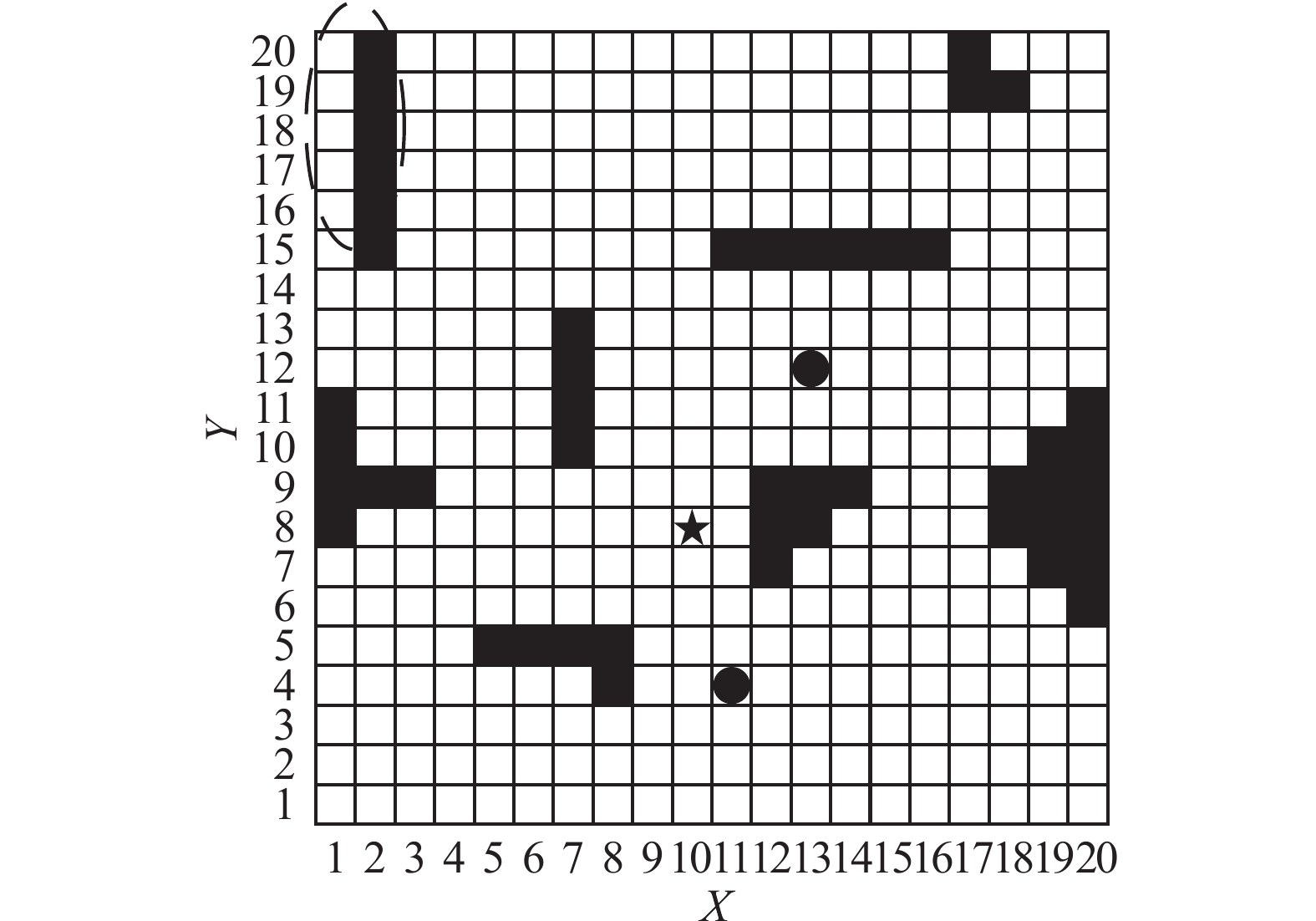Download: 图 4 多智能体覆盖环境 Fig. 4 The coverage environment of multi-agent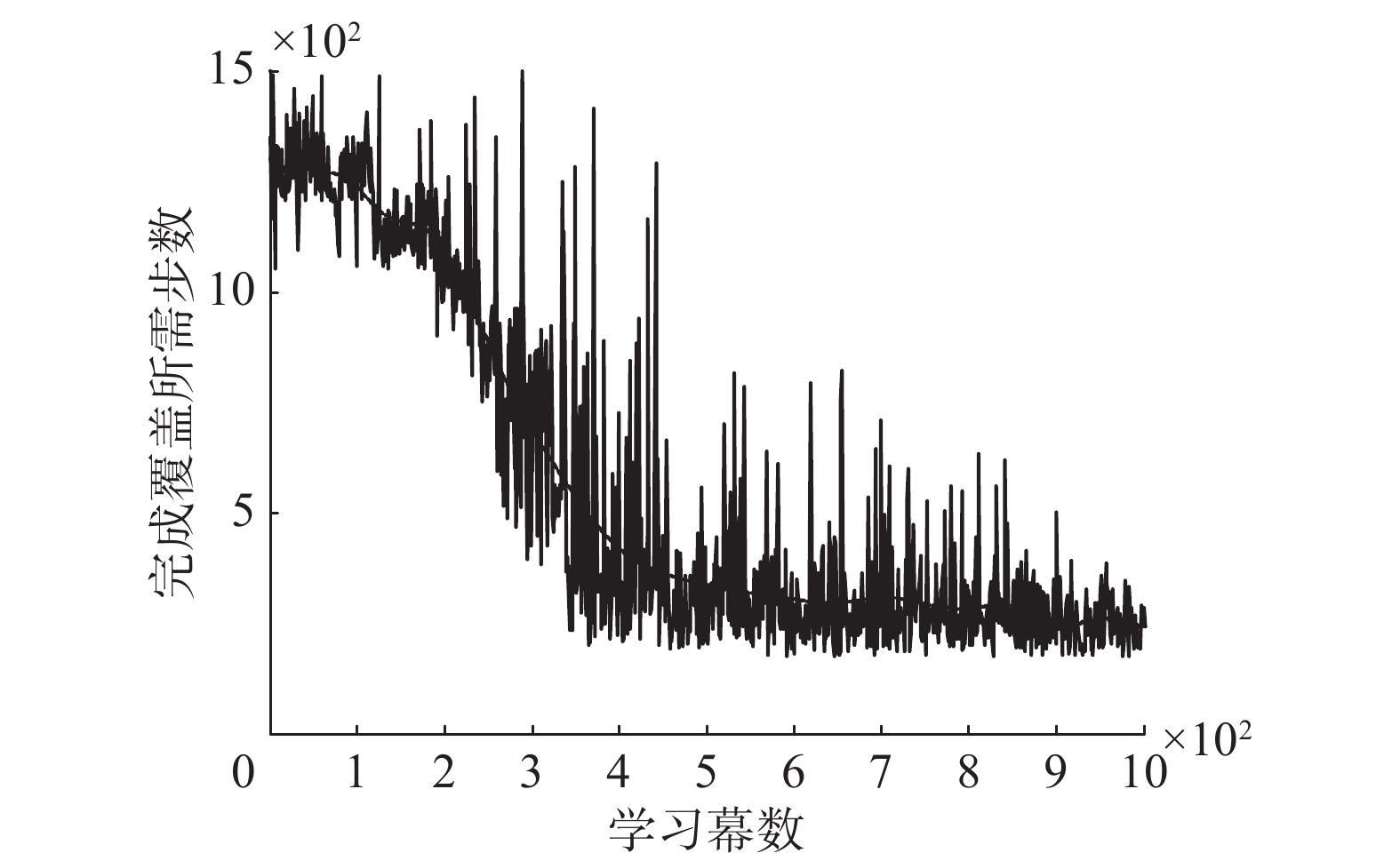Download: 图 5 异构多智能体覆盖完成步数 Fig. 5 The coverage steps of heterogeneous multi-agent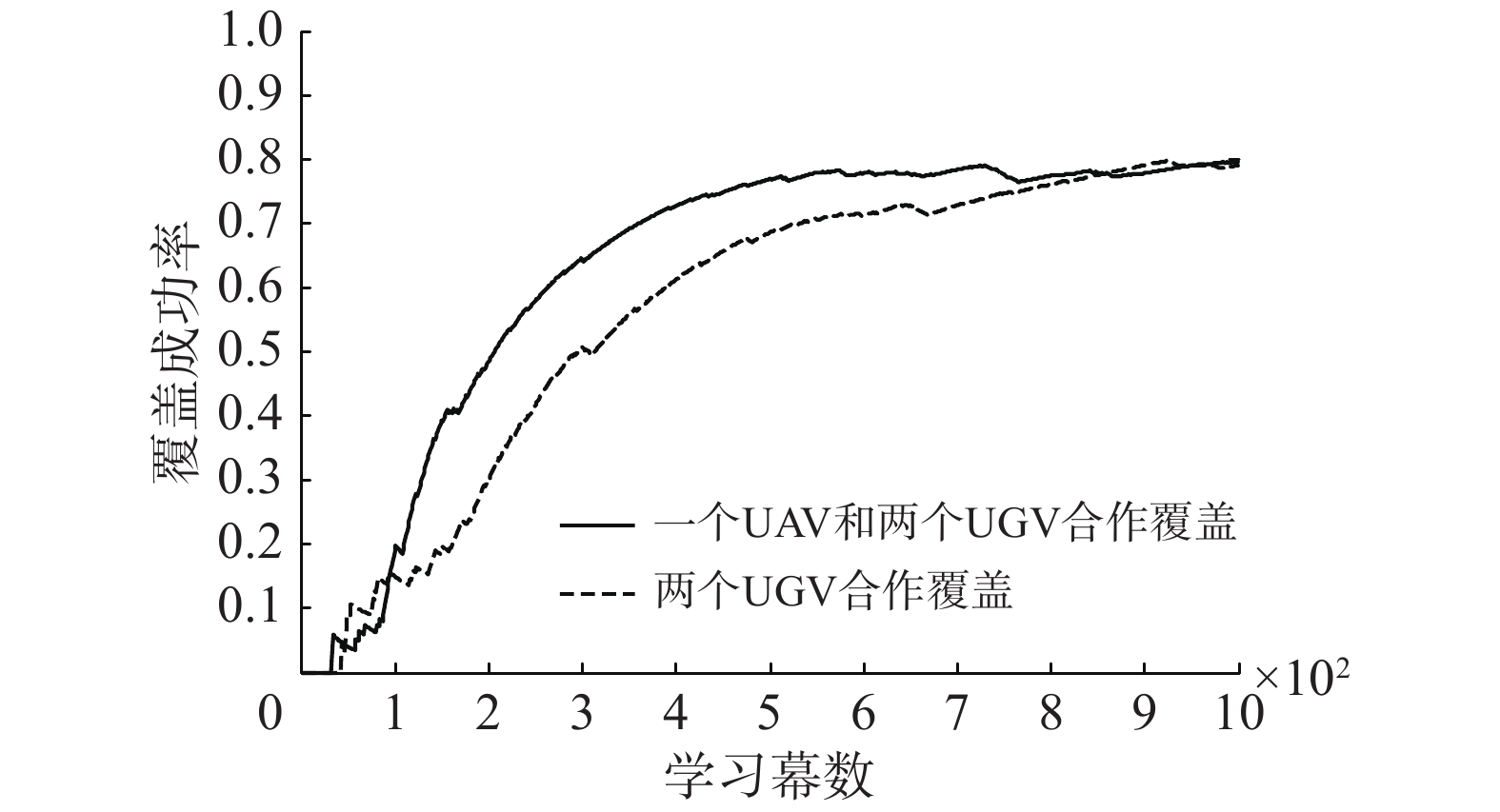Download: 图 6 覆盖试验成功率 Fig. 6 The success rate of coverage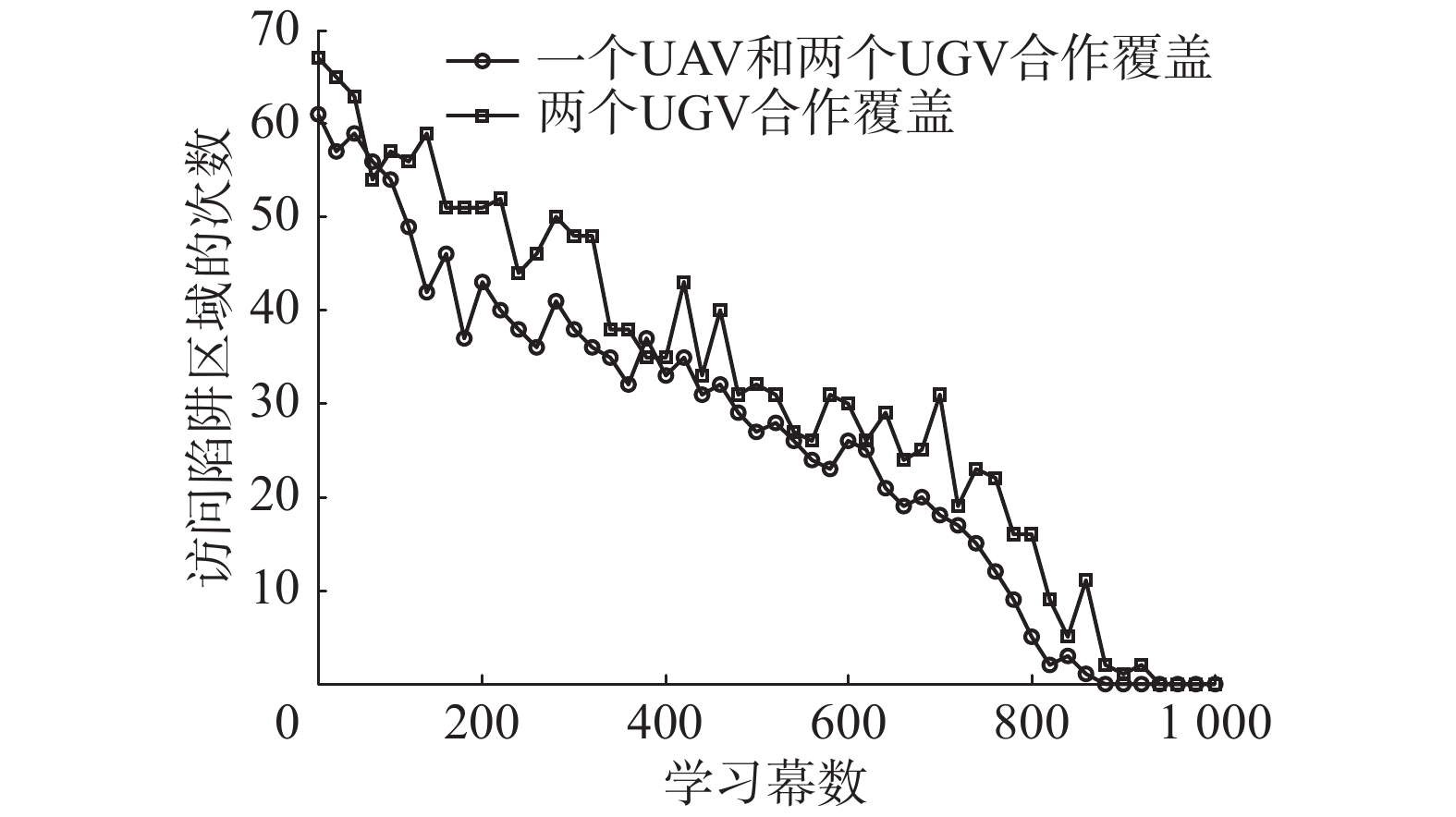Download: 图 7 陷阱区域访问次数统计 Fig. 7 The count of visits to the trap
5 结束语

  KANTAROS Y, ZAVLANOS M M. Distributed communication-aware coverage control by mobile sensor networks[J]. Automatica, 2016, 63: 209-220. DOI:10.1016/j.automatica.2015.10.035 (0)  蔡自兴, 崔益安. 多机器人覆盖技术研究进展[J]. 控制与决策, 2008, 23(5): 481-486, 491. CAI Zixing, CUI Yi’an. Survey of multi-robot coverage[J]. Control and decision, 2008, 23(5): 481-486, 491. (0)  MAHBOUBI H, MOEZZI K, AGHDAM A G, et al. Distributed deployment algorithms for improved coverage in a network of wireless mobile sensors[J]. IEEE transactions on industrial informatics, 2014, 10(1): 163-174. DOI:10.1109/TII.2013.2280095 (0)  TAO Dan, WU T Y. A survey on barrier coverage problem in directional sensor networks[J]. IEEE sensors journal, 2015, 15(2): 876-885. DOI:10.1109/JSEN.2014.2310180 (0)  TIAN Yuping, ZHANG Ya. High-order consensus of heterogeneous multi-agent systems with unknown communication delays[J]. Automatica, 2012, 48(6): 1205-1212. DOI:10.1016/j.automatica.2012.03.017 (0)  SONG Cheng, LIU Lu, FENG Gang, et al. Coverage control for heterogeneous mobile sensor networks on a circle[J]. Automatica, 2016, 63: 349-358. DOI:10.1016/j.automatica.2015.10.044 (0)  KANTAROS Y, THANOU M, TZES A. Distributed coverage control for concave areas by a heterogeneous robot-swarm with visibility sensing constraints[J]. Automatica, 2015, 53: 195-207. DOI:10.1016/j.automatica.2014.12.034 (0)  WANG Xinbing, HAN Sihui, WU Yibo, et al. Coverage and energy consumption control in mobile heterogeneous wireless sensor networks[J]. IEEE transactions on automatic control, 2013, 58(4): 975-988. DOI:10.1109/TAC.2012.2225511 (0)  SHARIFI F, CHAMSEDDINE A, MAHBOUBI H, et al. A distributed deployment strategy for a network of cooperative autonomous vehicles[J]. IEEE transactions on control systems technology, 2015, 23(2): 737-745. DOI:10.1109/TCST.2014.2341658 (0)  CHEN Jie, ZHANG Xing, XIN Bin, et al. Coordination between unmanned aerial and ground vehicles: a taxonomy and optimization perspective[J]. IEEE transactions on cybernetics, 2016, 46(4): 959-972. DOI:10.1109/TCYB.2015.2418337 (0)  ZHOU Yi, CHENG Nan, LU Ning, et al. Multi-UAV-aided networks: aerial-ground cooperative vehicular networking architecture[J]. IEEE vehicular technology magazine, 2015, 10(4): 36-44. DOI:10.1109/MVT.2015.2481560 (0)  PAPACHRISTOS C, TZES A. The power-tethered UAV-UGV team: a collaborative strategy for navigation in partially-mapped environments[C]//Proceedings of 22nd Mediterranean Conference of Control and Automation. Palermo, Italy, 2014: 1153–1158. (0)  GROCHOLSKY B, KELLER J, KUMAR V, et al. Cooperative air and ground surveillance[J]. IEEE robotics and automation magazine, 2006, 13(3): 16-25. DOI:10.1109/MRA.2006.1678135 (0)  KHALEGHI A M, XU Dong, WANG Zhenrui, et al. A DDDAMS-based planning and control framework for surveillance and crowd control via UAVs and UGVs[J]. Expert systems with applications, 2013, 40(18): 7168-7183. DOI:10.1016/j.eswa.2013.07.039 (0)  马磊, 张文旭, 戴朝华. 多机器人系统强化学习研究综述[J]. 西南交通大学学报, 2014, 49(6): 1032-1044. MA Lei, ZHANG Wenxu, DAI Chaohua. A review of developments in reinforcement learning for multi-robot systems[J]. Journal of southwest Jiaotong university, 2014, 49(6): 1032-1044. (0)  PUTERMAN M L. Markov decision processes: discrete stochastic dynamic programming[M]. New York: John Wiley and Sons, 1994. (0)  WATKINS C J C H, DAYAN P. Q-learning[J]. Machine learning, 1992, 8(3/4): 279-292. DOI:10.1023/A:1022676722315 (0)  WU Feng, ZILBERSTEIN S, CHEN Xiaoping. Online planning for multi-agent systems with bounded communication[J]. Artificial intelligence, 2011, 175(2): 487-511. DOI:10.1016/j.artint.2010.09.008 (0)